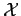# 2. 1 Numerical Discretization

The result of floating point numerical operations inevitably leads to a certain error, namely the numerical discretization error or truncation error. This error affects all calculations that are carried out with floating point arithmetic.

A necessary restriction on computational data types is that only a finite memory space can be used in order to represent a numeric value. This implies that only a finite number of numeric values can be represented while most of the numeric values of the solution can only be approximated. After a setof real numeric values is defined each real value that has to be treated numerically has to be approximated by members of the set. This inevitably results in a numerical truncation error which is made when the real values are replaced by members of the set.

Subsections

Michael 2008-01-16